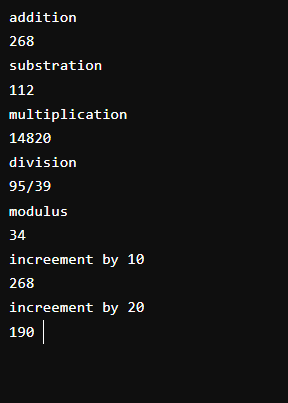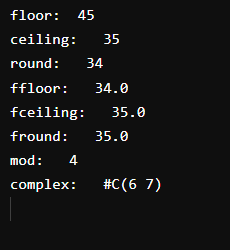# Numbers in LISP

• Last Updated : 08 Aug, 2022

LISP is a list processing programming language. It is widely used in the manipulation of data strings. It provides an input and output library. LISP provides a macro system and provides well control structures for the manipulation of data.

### LISP Math Function:

• floor:  floor returns the nearest smallest number.
• ceiling: ceiling return the nearest largest number.
• round: rounds the argument or quotient of the arguments to the nearest integer.
• mod: Returns the remainder in a division operation.
• complex:  Returns a number whose real part is real part and whose imaginary part is imaginary part

### Attributes Used:

• setq: It is used to set the value to a variable.
• printc: It is used for print output.
• terpri: It is used to produce a new line.
• write: It is used for writing.

Example 1:

## Lisp

 `; Normal Arithmetic calculation in``; LISP(Addition, Subtraction, Multiplication, division)``;set value ``1` `to ``190``; set value ``2` `to ``78``(setq val1 ``190``)``(setq val2 ``78``)``  ` `;addition operation``(princ ``"addition"``)``(print (``+` `val1 val2))` `;subtraction operation``(princ ``"substration"``)``(print (``-` `val1 val2))`` ` `;multiplication operation``(princ ``"multiplication"``)``(print (``*` `val1 val2))` `;division operation``(princ ``"division"``)``(print (``/` `val1 val2))` `;modulus operation``(princ ``"modulus"``)``(print (MOD val1 val2))` `;increment a by ``10``(princ ``"increment by 10"``)``(print (incf val1 val2))` `;decrement b by ``20``(princ ``"increment by 20"``)``(print (decf val1 val2))`

Output:Example 2:

## Lisp

 `;Predefined Arithmetic data type in LISP``(princ ``"floor:  "``)``(write (floor ``45.78``))``(terpri)` `(princ ``"ceiling:   "``)``(write (ceiling ``34.34``))``(terpri)` `(princ ``"round:   "``)``(write (round ``34.3``))``(terpri)` `(princ ``"ffloor:   "``)``(write (ffloor ``34.43``))``(terpri)` `(princ ``"fceiling:   "``)``(write (fceiling ``34.12``))``(terpri)` `(princ ``"fround:   "``)``(write (fround ``34.75``))``(terpri)` `(princ ``"mod:   "``)``(write (mod ``34` `5``))``(terpri)` `(princ ``"complex:   "``)``(setq c (complex ``6` `7``))``(write c)``(terpri)`

Output:My Personal Notes arrow_drop_up